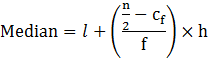Guru

# If the median of a distribution given below is 28.5 then, find the value of x & y. Q.2

• 0

Sir give me the best way to solve the problem of question from class 10th ncert question give me the best and easy way of question from statistics of exercise 14.3 of question no,1. give me the simple way of question If the median of a distribution given below is 28.5 then, find the value of x & y.

Share

1. Given data, n = 60

Median of the given data = 28.5

Where, n/2 = 30

Median class is 20 – 30 with a cumulative frequency = 25+x

Lower limit of median class, l = 20,

Cf = 5+x,

f = 20 & h = 10Substitute the values

28.5=20+((30−5−x)/20) × 10

8.5 = (25 – x)/2

17 = 25-x

Therefore, x =8

Now, from cumulative frequency, we can identify the value of x + y as follows:

Since,

60=5+20+15+5+x+y

Now, substitute the value of x, to find y

60 = 5+20+15+5+8+y

y = 60-53

y = 7

Therefore, the value of x = 8 and y = 7.

• 0